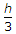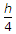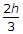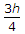# Civil Engineering - Applied Mechanics

46.
The c.g. of a thin hollow cone of height h, above its base lies on the axis, at a height ofExplanation:
No answer description is available. Let's discuss.

47.
Two forces act an angle of 120°. If the greater force is 50 kg and their resultant is perpendicular to the smaller force, the smaller force is
20 kg
25 kg
30 kg
35 kg
40 kg.
Explanation:
No answer description is available. Let's discuss.

48.
The C.G. of a right circular cone lies on its axis of symmetry at a height of
h/2
h/3
h/4
h/5
h/6.
Explanation:
No answer description is available. Let's discuss.

49.
A uniform rod 9 m long weighing 40 kg is pivoted at a point 2 m from one end where a weight of 120 kg is suspended. The required force acting at the end in a direction perpendicular to rod to keep it equilibrium, at an inclination 60° with horizontal, is
40 kg
60 kg
10 kg
100 kg.